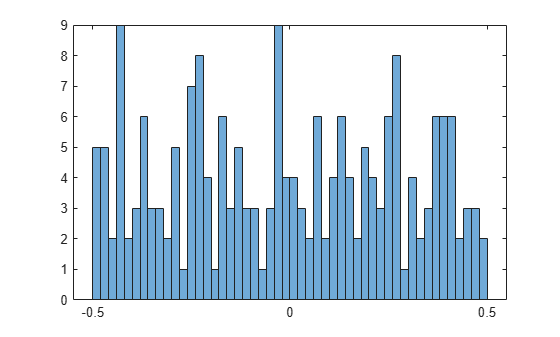Documentation

Numerical Computations With High Precision

This example shows how to use variable-precision arithmetic to obtain high precision computations using Symbolic Math Toolbox™.

Search for formulas that represent near-integers. A classic example is the following: compute $\mathrm{exp}\left(\sqrt{163}\cdot \pi \right)$ to 30 digits. The result appears to be an integer that is displayed with a rounding error.

digits(30);
f = exp(sqrt(sym(163))*sym(pi));
vpa(f)
ans = $262537412640768743.999999999999$

Compute the same value to 40 digits. It turns out that this is not an integer.

digits(40);
vpa(f)
ans = $262537412640768743.9999999999992500725972$

Investigate this phenomenon further. Below, numbers up to $\mathrm{exp}\left(1000\right)$ occur, and the investigation needs some correct digits after the decimal point. Compute the required working precision:

d = log10(exp(vpa(1000)))
d = $434.2944819032518276511289189166050822944$

Set the required precision before the first call to a function that depends on it. Among others, round, vpa, and double are such functions.

digits(ceil(d) + 50);

Look for similar examples of the form $\mathrm{exp}\phantom{\rule{-0.16666666666666666em}{0ex}}\left(\sqrt{n}\pi \right)$. Of course, you can obtain more such numbers n by multiplying 163 by a square. But apart from that, many more numbers of this form are close to some integer. You can see this from a histogram plot of their fractional parts:

A = exp(pi*sqrt(vpa(1:1000)));
B = A-round(A);
histogram(double(B), 50)Calculate if there are near-integers of the form $\mathrm{exp}\left(n\right)$.

A = exp(vpa(1:1000));
B = A-round(A);
find(abs(B) < 1/1000)
ans =

1x0 empty double row vector

It turns out that this time the fractional parts of the elements of A are rather evenly distributed.

histogram(double(B), 50)Mathematical Modeling with Symbolic Math Toolbox

Get examples and videos